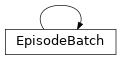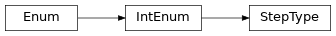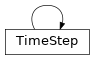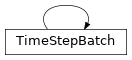# garage._dtypes¶

Data types for agent-based learning.

class EpisodeBatch

Bases: collections.namedtuple()A tuple representing a batch of whole episodes.

Data type for on-policy algorithms.

A EpisodeBatch represents a batch of whole episodes, produced when one or more agents interacts with one or more environments.

Symbol

Description

$$N$$

Episode batch dimension

$$[T]$$

Variable-length time dimension of each episode

$$S^*$$

Single-step shape of a time-series tensor

$$N \bullet [T]$$

A dimension computed by flattening a variable-length time dimension $$[T]$$ into a single batch dimension with length $$sum_{i \in N} [T]_i$$

env_spec

Specification for the environment from which this data was sampled.

Type

EnvSpec

observations

A numpy array of shape $$(N \bullet [T], O^*)$$ containing the (possibly multi-dimensional) observations for all time steps in this batch. These must conform to EnvStep.observation_space.

Type

numpy.ndarray

last_observations

A numpy array of shape $$(N, O^*)$$ containing the last observation of each episode. This is necessary since there are one more observations than actions every episode.

Type

numpy.ndarray

actions

A numpy array of shape $$(N \bullet [T], A^*)$$ containing the (possibly multi-dimensional) actions for all time steps in this batch. These must conform to EnvStep.action_space.

Type

numpy.ndarray

rewards

A numpy array of shape $$(N \bullet [T])$$ containing the rewards for all time steps in this batch.

Type

numpy.ndarray

env_infos

A dict of numpy arrays arbitrary environment state information. Each value of this dict should be a numpy array of shape $$(N \bullet [T])$$ or $$(N \bullet [T], S^*)$$.

Type

dict

agent_infos

A dict of numpy arrays arbitrary agent state information. Each value of this dict should be a numpy array of shape $$(N \bullet [T])$$ or $$(N \bullet [T], S^*)$$. For example, this may contain the hidden states from an RNN policy.

Type

numpy.ndarray

step_types

A numpy array of StepType with shape :math:(N,) containing the time step types for all transitions in this batch.

Type

numpy.ndarray

lengths

An integer numpy array of shape $$(N,)$$ containing the length of each episode in this batch. This may be used to reconstruct the individual episodes.

Type

numpy.ndarray

Raises

ValueError – If any of the above attributes do not conform to their prescribed types and shapes.

classmethod concatenate(cls, *batches)[source]

Create a EpisodeBatch by concatenating EpisodeBatches.

Parameters

batches (list[EpisodeBatch]) – Batches to concatenate.

Returns

The concatenation of the batches.

Return type

EpisodeBatch

split(self)[source]

Split an EpisodeBatch into a list of EpisodeBatches.

The opposite of concatenate.

Returns

A list of EpisodeBatches, with one

episode per batch.

Return type
to_list(self)[source]

Convert the batch into a list of dictionaries.

Returns

Keys:
• observations (np.ndarray): Non-flattened array of

observations. Has shape (T, S^*) (the unflattened state space of the current environment). observations[i] was used by the agent to choose actions[i].

• next_observations (np.ndarray): Non-flattened array of

observations. Has shape (T, S^*). next_observations[i] was observed by the agent after taking actions[i].

• actions (np.ndarray): Non-flattened array of actions. Should

have shape (T, S^*) (the unflattened action space of the current environment).

• rewards (np.ndarray): Array of rewards of shape (T,) (1D

array of length timesteps).

• agent_infos (dict[str, np.ndarray]): Dictionary of stacked,

non-flattened agent_info arrays.

• env_infos (dict[str, np.ndarray]): Dictionary of stacked,

non-flattened env_info arrays.

• step_types (numpy.ndarray): A numpy array of StepType with

shape (T,) containing the time step types for all transitions in this batch.

Return type

list[dict[str, np.ndarray or dict[str, np.ndarray]]]

classmethod from_list(cls, env_spec, paths)[source]

Create a EpisodeBatch from a list of episodes.

Parameters
• env_spec (EnvSpec) – Specification for the environment from which this data was sampled.

• paths (list[dict[str, np.ndarray or dict[str, np.ndarray]]]) –

Keys: * observations (np.ndarray): Non-flattened array of

observations. Typically has shape (T, S^*) (the unflattened state space of the current environment). observations[i] was used by the agent to choose actions[i]. observations may instead have shape (T + 1, S^*).

• next_observations (np.ndarray): Non-flattened array of

observations. Has shape (T, S^*). next_observations[i] was observed by the agent after taking actions[i]. Optional. Note that to ensure all information from the environment was preserved, observations[i] should have shape (T + 1, S^*), or this key should be set. However, this method is lenient and will “duplicate” the last observation if the original last observation has been lost.

• actions (np.ndarray): Non-flattened array of actions. Should

have shape (T, S^*) (the unflattened action space of the current environment).

• rewards (np.ndarray): Array of rewards of shape (T,) (1D

array of length timesteps).

• agent_infos (dict[str, np.ndarray]): Dictionary of stacked,

non-flattened agent_info arrays.

• env_infos (dict[str, np.ndarray]): Dictionary of stacked,

non-flattened env_info arrays.

• step_types (numpy.ndarray): A numpy array of StepType with

shape (T,) containing the time step types for all transitions in this batch.

property next_observations(self)

Get the observations seen after actions are performed.

Usually, in an EpisodeBatch, next_observations don’t need to be stored explicitly, since the next observation is already stored in the batch.

Returns

The “next_observations”.

Return type

np.ndarray

property padded_observations(self)

Returns

$$(N, max_episode_length, O^*)$$.

Return type

np.ndarray

property padded_actions(self)

Returns

$$(N, max_episode_length, A^*)$$.

Return type

np.ndarray

property observations_list(self)

Split observations into a list.

Returns

Splitted list.

Return type

list[np.ndarray]

property actions_list(self)

Split actions into a list.

Returns

Splitted list.

Return type

list[np.ndarray]

property padded_rewards(self)

Returns

$$(N, max_episode_length)$$.

Return type

np.ndarray

property valids(self)

An array indicating valid steps in a padded tensor.

Returns

the shape is $$(N, max_episode_length)$$.

Return type

np.ndarray

property padded_agent_infos(self)

Returns

Padded agent infos. Each value should have

shape with $$(N, max_episode_length)$$ or $$(N, max_episode_length, S^*)$$.

Return type

dict[str, np.ndarray]

pad_to_last(self, input_array)[source]

Parameters

input_array (np.ndarray) – Tensors to be padded.

Returns

$$(N, max_episode_length)$$ or $$(N, max_episode_length, S^*)$$.

Return type

numpy.ndarray

count()

Return number of occurrences of value.

index()

Return first index of value.

Raises ValueError if the value is not present.

class StepType[source]

Bases: enum.IntEnumDefines the status of a TimeStep within a sequence.

Note that the last TimeStep in a sequence can either be :attribute:StepType.TERMINAL or :attribute:StepType.TIMEOUT.

Suppose max_episode_length = 5: * A success sequence terminated at step 4 will look like:

FIRST, MID, MID, TERMINAL

• A success sequence terminated at step 5 will look like:

FIRST, MID, MID, MID, TERMINAL

• An unsuccessful sequence truncated by time limit will look like:

FIRST, MID, MID, MID, TIMEOUT

class denominator

the denominator of a rational number in lowest terms

class imag

the imaginary part of a complex number

class numerator

the numerator of a rational number in lowest terms

class real

the real part of a complex number

FIRST = 0
MID = 1
TERMINAL = 2
TIMEOUT = 3
classmethod get_step_type(cls, step_cnt, max_episode_length, done)[source]

Determines the step type based on step cnt and done signal.

Parameters
• step_cnt (int) – current step cnt of the environment.

• max_episode_length (int) – maximum episode length.

• done (bool) – the done signal returned by Environment.

Returns

the step type.

Return type

StepType

Raises
• ValueError – if step_cnt is < 1. In this case a environment’s

• reset() is likely not called yet and the step_cnt is None.

bit_length()

Number of bits necessary to represent self in binary.

>>> bin(37)
'0b100101'
>>> (37).bit_length()
6

conjugate()

Returns self, the complex conjugate of any int.

to_bytes()

Return an array of bytes representing an integer.

length

Length of bytes object to use. An OverflowError is raised if the integer is not representable with the given number of bytes.

byteorder

The byte order used to represent the integer. If byteorder is ‘big’, the most significant byte is at the beginning of the byte array. If byteorder is ‘little’, the most significant byte is at the end of the byte array. To request the native byte order of the host system, use sys.byteorder’ as the byte order value.

signed

Determines whether two’s complement is used to represent the integer. If signed is False and a negative integer is given, an OverflowError is raised.

name(self)

The name of the Enum member.

value(self)

The value of the Enum member.

class TimeStep[source]

Bases: collections.namedtuple()A tuple representing a single TimeStep.

A TimeStep represents a single sample when an agent interacts

with an environment. It describes as SARS (State–action–reward–state) tuple that characterizes the evolution of a MDP.

env_spec

Specification for the environment from which this data was sampled.

Type

EnvSpec

observation

A numpy array of shape $$(O^*)$$ containing the observation for the this time step in the environment. These must conform to EnvStep.observation_space. The observation before applying the action. None if step_type is StepType.FIRST, i.e. at the start of a sequence.

Type

numpy.ndarray

action

A numpy array of shape $$(A^*)$$ containing the action for the this time step. These must conform to EnvStep.action_space. None if step_type is StepType.FIRST, i.e. at the start of a sequence.

Type

numpy.ndarray

reward

A float representing the reward for taking the action given the observation, at the this time step. None if step_type is StepType.FIRST, i.e. at the start of a sequence.

Type

float

next_observation

A numpy array of shape $$(O^*)$$ containing the observation for the this time step in the environment. These must conform to EnvStep.observation_space. The observation after applying the action.

Type

numpy.ndarray

env_info

A dict arbitrary environment state information.

Type

dict

agent_info

A dict of arbitrary agent state information. For example, this may contain the hidden states from an RNN policy.

Type

dict

step_type
Type

StepType

property first(self)

bool: Whether this step is the first of its episode.

property mid(self)

bool: Whether this step is in the middle of its episode.

property terminal(self)

bool: Whether this step records a termination condition.

property timeout(self)

bool: Whether this step records a timeout condition.

property last(self)

bool: Whether this step is the last of its episode.

classmethod from_env_step(cls, env_step, last_observation, agent_info)[source]

Create a TimeStep from a EnvStep.

Parameters
• env_step (EnvStep) – the env step returned by the environment.

• last_observation (numpy.ndarray) – A numpy array of shape $$(O^*)$$ containing the observation for the this time step in the environment. These must conform to EnvStep.observation_space. The observation before applying the action.

• agent_info (dict) – A dict of arbitrary agent state information.

Returns

The TimeStep with all information of EnvStep plus the agent info.

Return type

TimeStep

count()

Return number of occurrences of value.

index()

Return first index of value.

Raises ValueError if the value is not present.

class InOutSpec(input_space, output_space)[source]

Describes the input and output spaces of a primitive or module.

Parameters
• input_space (akro.Space) – Input space of a module.

• output_space (akro.Space) – Output space of a module.

property input_space(self)

Get input space of the module.

Returns

Input space of the module.

Return type

akro.Space

property output_space(self)

Get output space of the module.

Returns

Output space of the module.

Return type

akro.Space

class TimeStepBatch[source]

Bases: collections.namedtuple()A tuple representing a batch of TimeSteps.

Data type for off-policy algorithms, imitation learning and batch-RL.

env_spec

Specification for the environment from which this data was sampled.

Type

EnvSpec

observations

Non-flattened array of observations. Typically has shape (batch_size, S^*) (the unflattened state space of the current environment).

Type

numpy.ndarray

actions

Non-flattened array of actions. Should have shape (batch_size, S^*) (the unflattened action space of the current environment).

Type

numpy.ndarray

rewards

Array of rewards of shape (batch_size, 1).

Type

numpy.ndarray

next_observation

Non-flattened array of next observations. Has shape (batch_size, S^*). next_observations[i] was observed by the agent after taking actions[i].

Type

numpy.ndarray

env_infos

A dict arbitrary environment state information.

Type

dict

agent_infos

A dict of arbitrary agent state information. For example, this may contain the hidden states from an RNN policy.

Type

dict

step_types

A numpy array of StepType with shape ( batch_size,) containing the time step types for all transitions in this batch.

Type

numpy.ndarray

Raises

ValueError – If any of the above attributes do not conform to their prescribed types and shapes.

classmethod concatenate(cls, *batches)[source]

Concatenate two or more :class:TimeStepBatchs.

Parameters

batches (list[TimeStepBatch]) – Batches to concatenate.

Returns

The concatenation of the batches.

Return type

TimeStepBatch

Raises

ValueError – If no TimeStepBatches are provided.

split(self)[source]

Split a TimeStepBatch into a list of :class:~TimeStepBatchs.

The opposite of concatenate.

Returns

A list of :class:TimeStepBatchs, with one

Return type
to_time_step_list(self)[source]

Convert the batch into a list of dictionaries.

Breaks the TimeStepBatch into a list of single time step sample dictionaries. len(rewards) (or the number of discrete time step) dictionaries are returned

Returns

Keys:
observations (numpy.ndarray): Non-flattened array of

observations. Typically has shape (batch_size, S^*) (the unflattened state space of the current environment).

actions (numpy.ndarray): Non-flattened array of actions. Should

have shape (batch_size, S^*) (the unflattened action space of the current environment).

rewards (numpy.ndarray): Array of rewards of shape (

batch_size,) (1D array of length batch_size).

next_observation (numpy.ndarray): Non-flattened array of next

observations. Has shape (batch_size, S^*). next_observations[i] was observed by the agent after taking actions[i].

env_infos (dict): A dict arbitrary environment state

information.

agent_infos (dict): A dict of arbitrary agent state

information. For example, this may contain the hidden states from an RNN policy.

step_types (numpy.ndarray): A numpy array of StepType with

shape (batch_size,) containing the time step types for all transitions in this batch.

Return type

list[dict[str, np.ndarray or dict[str, np.ndarray]]]

property terminals(self)

Get an array of boolean indicating ternianal information.

Returns

An array of boolean of shape (batch_size, 1)

indicating whether the StepType is TERMINAL

Return type

numpy.ndarray

classmethod from_time_step_list(cls, env_spec, ts_samples)[source]

Create a TimeStepBatch from a list of time step dictionaries.

Parameters
• env_spec (EnvSpec) – Specification for the environment from which this data was sampled.

• ts_samples (list[dict[str, np.ndarray or dict[str, np.ndarray]]]) –

keys: * observations (numpy.ndarray): Non-flattened array of

observations. Typically has shape (batch_size, S^*) (the unflattened state space of the current environment).

• actions (numpy.ndarray): Non-flattened array of actions.

Should have shape (batch_size, S^*) (the unflattened action space of the current environment).

• rewards (numpy.ndarray): Array of rewards of shape (

batch_size,) (1D array of length batch_size).

• next_observation (numpy.ndarray): Non-flattened array of next

observations. Has shape (batch_size, S^*). next_observations[i] was observed by the agent after taking actions[i].

• env_infos (dict): A dict arbitrary environment state

information.

• agent_infos (dict): A dict of arbitrary agent

state information. For example, this may contain the hidden states from an RNN policy.

• step_types (numpy.ndarray): A numpy array of StepType with

shape (batch_size,) containing the time step types for all

transitions in this batch.

Returns

The concatenation of samples.

Return type

TimeStepBatch

Raises

ValueError – If no dicts are provided.

classmethod from_episode_batch(cls, batch)[source]

Construct a TimeStepBatch from an EpisodeBatch.

Parameters

batch (EpisodeBatch) – Episode batch to convert.

Returns

The converted batch.

Return type

TimeStepBatch

count()

Return number of occurrences of value.

index`()

Return first index of value.

Raises ValueError if the value is not present.